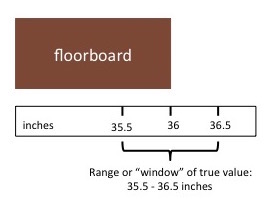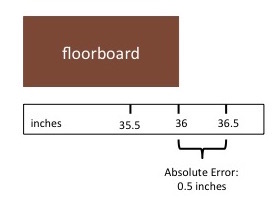# Absolute & Relative Error: Definition & Formula

Lesson Transcript
Instructor: Catherine Glover

Catherine has a master's degree in Mathematical Biology and teaches math at the college level.

Did you know that measuring tools are not 100% accurate? Read this lesson to learn more about the accuracy of measuring tools and how this influences the absolute and relative error of measurements.

## Fitting In

Dan has a 36-inch-long floorboard in his living room that needs to be replaced. He goes to the store to purchase a new floorboard, which he measures at exactly 36 inches long, but when he tries to install it in his floor, the new floorboard is about half an inch too long. What's going on here?

Dan didn't make a mistake. It turns out that the tools we use to measure properties of objects, like their size or weight, are not completely accurate. They are mostly accurate, but there's always some error in any tool of measurement. In this lesson, we'll talk about the accuracy of measuring tools and how this influences two types of error.An error occurred trying to load this video.

Try refreshing the page, or contact customer support.

Coming up next: How to Write Sets Using Set Builder Notation

### You're on a roll. Keep up the good work!

Replay
Your next lesson will play in 10 seconds
• 0:04 Fitting In
• 0:42 Measuring Tools & Accuracy
• 1:20 Absolute Error
• 2:18 Relative Error
• 3:25 Comparing Relative Errors
• 5:10 Lesson Summary
Save Save

Want to watch this again later?

Timeline
Autoplay
Autoplay
Speed Speed

## Measuring Tools & Accuracy

Tools of measurement are said to be accurate to a certain degree. Let's say that the measuring tape Dan used to measure his new floorboard was accurate to 1 inch. This means that there is a 1-inch range, or ''window'', around a measurement where the true value could actually be located. From the measured value, that's half an inch on either side. Dan measured the new floorboard at 36 inches long, but the true length could be anywhere between 35.5 inches and 36.5 inches long. In this case, the floorboard was actually on the longer end. We say that the measurement is 36 ± 0.5 inches.## Absolute Error

There are two types of error that are affected by the accuracy of measuring tools. The absolute error is defined as the absolute value (or magnitude) of the difference between the measured value and the true value. Thus, let:

• ea = the absolute error
• xm = the measured value
• xt = the true value

The formula for computing absolute error is:

ea = | xm - xt |

There's only one catch - we don't usually know what the true value is. In that case, we just need to think about the accuracy of the measuring tool. Let's use Dan's measuring tape as an example. It is accurate to 1 inch, which means that the true value of any measurement could be up to half an inch smaller or half an inch larger than the actual measurement. The maximum possible difference between the measurement and its true value is half an inch. Therefore, the absolute error is 0.5 inches.## Relative Error

There is another type of error that is affected by the accuracy of measuring tools. The relative error is defined as the absolute error relative to the size of the measurement. All you need to do is divide the absolute error by the measured value. In addition to the variables, let:

• er = the relative error

Then the formula for computing relative error is:

• er = ea / xm

In Dan's situation, the absolute error is 0.5 inches, and he measured the floorboard at 36 inches long, so the relative error is:

er = 0.5 inches/36 inches = 0.014 (rounded to the nearest thousandth)

The relative error doesn't have units because they cancel out during the calculation. The relative error is a proportion, so we can also express it as a percentage by multiplying the relative error by 100%, like this:

er = 0.014 * 100% = 1.4%

We can say that the absolute error is 1.4% of the measured value.

To unlock this lesson you must be a Study.com Member.

### Register to view this lesson

Are you a student or a teacher?